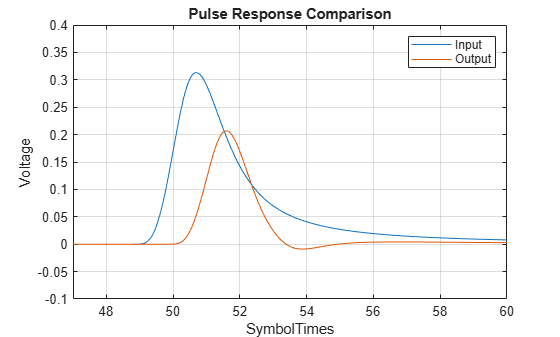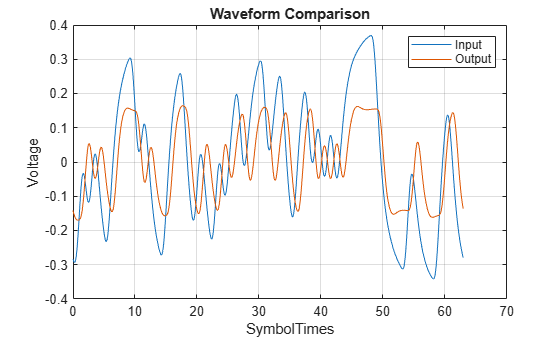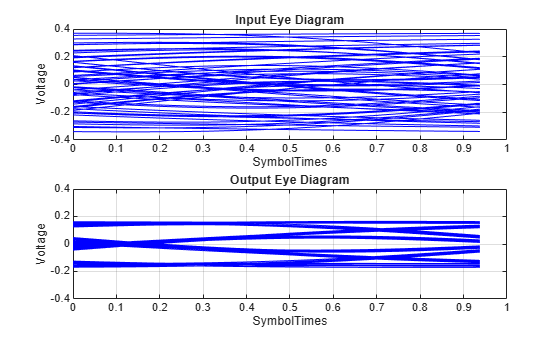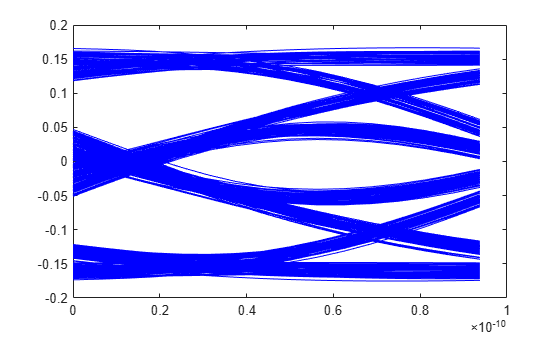# serdes.FFE

Models a feed-forward equalizer

## Description

The `serdes.FFE` System object™ applies a feed-forward equalizer (FFE) as a symbol-spaced finite-impulse response (FIR) filter. Apply the equalizer to a sample-by-sample input signal or an impulse response vector input signal to reduce distortions due to channel loss impairments.

To equalize the baseband signal:

1. Create the `serdes.FFE` object and set its properties.

2. Call the object with arguments, as if it were a function.

## Creation

### Syntax

``ffe = serdes.FFE``
``ffe = serdes.FFE(Name,Value)``

### Description

````ffe = serdes.FFE` returns an FFE object that modifies an input waveform according to the finite impulse response (FIR) transfer function defined in the object.```
````ffe = serdes.FFE(Name,Value)` sets properties using one or more name-value pairs. Enclose each property name in quotes. Unspecified properties have default values.Example: `ffe = serdes.FFE('Mode',1)` returns an FFE object that applies specified tap weights to the input waveform.```

## Properties

expand all

Unless otherwise indicated, properties are nontunable, which means you cannot change their values after calling the object. Objects lock when you call them, and the `release` function unlocks them.

If a property is tunable, you can change its value at any time.

### Main

FFE operating mode, specified as `0` or `1`. `Mode` determines whether FFE is bypassed or not.

Mode ValueFFE ModeFFE Operation
`0`Off`serdes.FFE` is bypassed and the input waveform remains unchanged.
`1`Fixed`serdes.FFE` applies input FFE tap weights, specified in `TapWeights`, to input waveform.

Data Types: `double`

FFE tap weights, specified as a row vector in V. The length of the vector specifies the number of taps. Each vector element's value specifies the strength of the tap at that position. The tap with the largest magnitude is the main tap and therefore defines the number of pre- and post-cursor taps.

Data Types: `double`

Normalize tap weight vectors, specified as `true` or `false`. When set to `true`, the object scales the `TapWeights` vector elements so that the sum of their absolute values is 1.

Data Types: `logical`

Time of a single symbol duration, specified as a real scalar in s.

Data Types: `double`

Uniform time step of the waveform, specified as a real scalar in s.

Data Types: `double`

Input waveform type:

• `'Sample'` — A sample-by-sample input signal

• `'Impulse'` — An impulse response input signal

Data Types: `char`

## Usage

### Syntax

``y = ffe(x)``

### Description

``y = ffe(x)``

### Input Arguments

expand all

Input baseband signal. If the `WaveType` is set to `'Sample'`, the input signal is a sample-by-sample signal specified as a scalar. If the `WaveType` is set to `'Impulse'`, the input signal must be an impulse response vector signal.

### Output Arguments

expand all

Filtered channel output. If the input signal is a sample-by-sample signal specified as a scalar, the output is also scalar. If the input signal is an impulse response vector signal, the output is also a vector.

## Object Functions

To use an object function, specify the System object as the first input argument. For example, to release system resources of a System object named `obj`, use this syntax:

`release(obj)`

expand all

 `step` Run System object algorithm `release` Release resources and allow changes to System object property values and input characteristics `reset` Reset internal states of System object

## Examples

collapse all

This example shows how to process impulse response of a channel using `serdes.FFE` System object™.

Use a symbol time of 100 ps and 16 samples per symbol. The channel has 16 dB loss.

```SymbolTime = 100e-12; SamplesPerSymbol = 16; dbloss = 16; ```

Calculate the sample interval.

`dt = SymbolTime/SamplesPerSymbol;`

Create the FFE object with fixed mode of operation.

```TapWeights = [0 0.7 -0.2 -0.10]; FFEMode = 1; FFE1 = serdes.FFE('SymbolTime',SymbolTime,'SampleInterval',dt,... 'Mode',FFEMode,'WaveType','Impulse',... 'TapWeights',TapWeights);```

Create the channel impulse response.

```channel = serdes.ChannelLoss('Loss',dbloss,'dt',dt,... 'TargetFrequency',1/SymbolTime/2); impulseIn = channel.impulse;```

Process the impulse response with FFE.

`impulseOut = FFE1(impulseIn);`

Convert the impulse responses to pulse, waveform and eye diagram data for visualization in later steps. Initialize the pseudorandom binary sequence (PRBS).

```ord = 6; dataPattern = prbs(ord,2^ord-1)-0.5; pulseIn = impulse2pulse(impulseIn,SamplesPerSymbol,dt); waveIn = pulse2wave(pulseIn,dataPattern,SamplesPerSymbol); eyeIn = reshape(waveIn,SamplesPerSymbol,[]); pulseOut = impulse2pulse(impulseOut,SamplesPerSymbol,dt); waveOut = pulse2wave(pulseOut,dataPattern,SamplesPerSymbol); eyeOut = reshape(waveOut,SamplesPerSymbol,[]);```

Create the time vectors.

```t = dt*(0:length(pulseOut)-1)/SymbolTime; teye = t(1:SamplesPerSymbol); t2 = dt*(0:length(waveOut)-1)/SymbolTime;```

Plot the pulse response comparison, waveform comparison, and input and output eye diagrams.

```figure plot(t,pulseIn,t,pulseOut) legend('Input','Output') title('Pulse Response Comparison') xlabel('SymbolTimes'),ylabel('Voltage') grid on axis([47 60 -0.1 0.4])``````figure plot(t2,waveIn,t2,waveOut) legend('Input','Output') title('Waveform Comparison') xlabel('SymbolTimes') ylabel('Voltage') grid on ``````figure subplot(211),plot(teye,eyeIn,'b') ax = axis; xlabel('SymbolTimes') ylabel('Voltage') grid on title('Input Eye Diagram') subplot(212),plot(teye,eyeOut,'b') axis(ax); xlabel('SymbolTimes') ylabel('Voltage') grid on title('Output Eye Diagram')```This example shows how to process impulse response of a channel one sample at a time using `serdes.FFE` System object™.

Use a symbol time of 100 ps with 16 samples per symbol. The channel has 16 dB loss.

```SymbolTime = 100e-12; SamplesPerSymbol = 16; dbloss = 16; ```

Calculate the sample interval.

`dt = SymbolTime/SamplesPerSymbol;`

Create the FFE object with fixed mode.

```FFEMode = 1; TapWeights = [0 0.7 -0.2 -0.1]; FFE = serdes.FFE('SymbolTime',SymbolTime,'SampleInterval',dt,... 'Mode',FFEMode,'WaveType','Sample',... 'TapWeights',TapWeights);```

Create the channel impulse response.

```channel = serdes.ChannelLoss('Loss',dbloss,'dt',dt,... 'TargetFrequency',1/SymbolTime/2);```

Create the eye diagram.

```eyediagram = comm.EyeDiagram('SampleRate',1/dt,'SamplesPerSymbol',SamplesPerSymbol,... 'YLimits',[-0.5 0.5]);```

Initialize the pseudorandom binary sequence (PRBS) code generator of order 12.

```prbsOrder = 12; M = 500; %number of symbols to simulate [dataBit,prbsSeed]=prbs(prbsOrder,1);```

Loop through one symbol at a time.

```inwave = zeros(SamplesPerSymbol,1); outwave = zeros(SamplesPerSymbol,1); for ii = 1:M %Get new symbol [dataBit,prbsSeed]=prbs(prbsOrder,1,prbsSeed); inwave(1:SamplesPerSymbol) = dataBit-0.5; %convolve input waveform with channel y = channel(inwave); %process one sample at a time through the FFE for jj = 1:SamplesPerSymbol outwave(jj) = FFE(y(jj)); end %Plot eye diagram eyediagram(outwave) end```## Extended Capabilities